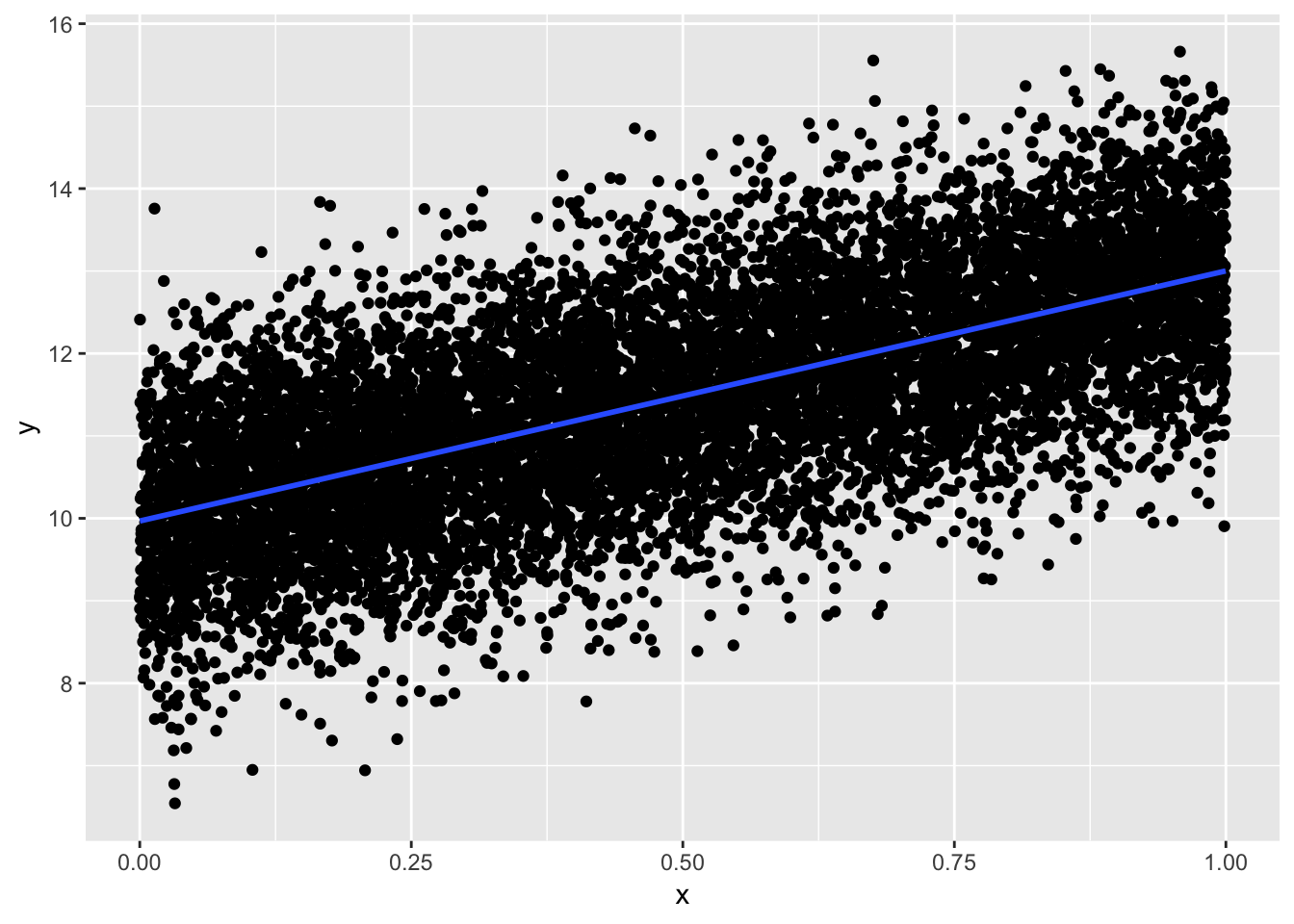## Pathological examples of model condition violations

Because most real models are hard to assess, we want to examine some pathological examples of the LINE conditions for regression being violated.

For all these examples, we will generate simulated data to show the particular conditions being violated.

You’ll notice that even as we’re trying to simulate data to violate one condition, the others start to look bad, too. This is very common in the real world, as well!

#### Good Model

First, a positive example! Let’s make some good data.

``````n = 10000
beta0 = 10
beta1 = 3
x = runif(n)
e = rnorm(n)
ds = data.frame(y = beta0 + beta1 * x + e)``````

Now, we can look at the relationship and check the conditions.

``ggplot(ds, aes(x=x, y=y)) + geom_point() + stat_smooth(method=lm, se=FALSE)````````mod = lm(y ~ x, data=ds)
plot(mod, which=1)``````